# How to Create Pie Chart from Pandas DataFrame?

• Last Updated : 19 Dec, 2021

In this article, we will discuss how to create a Pie chart from Pandas dataframe using Python.

The data in a circular graph is represented by a pie chart, which is a form of a graph. In research, engineering, and business, it is frequently utilized. The segments of the pie depict the data’s relative strength and are a sort of graphical representation of data. A list of categories and numerical variables is required for a pie chart. The phrase “pie” refers to the entire, whereas “slices” refers to the individual components of the pie. It is divided into segments and sectors, with each segment and sector representing a piece of the whole pie chart (percentage). All of the data adds up to 360 degrees. The pie’s entire worth is always 100 percent.

Let us first create a simple Pie chart.

## Simple Pie chart

For this first, all required modules are imported and a dataframe is initialized. To plot a pie chart plot() function is used and the kind attribute is set to pie.

Syntax:

`plot(kind='pie')`

Example: A simple pie chart

## Python3

 `import` `pandas as pd`` ` `# DataFrame of each student and the votes they get``dataframe ``=` `pd.DataFrame({``'Name'``: [``'Aparna'``, ``'Aparna'``, ``'Aparna'``,``                                   ``'Aparna'``, ``'Aparna'``, ``'Juhi'``, ``                                   ``'Juhi'``, ``'Juhi'``, ``'Juhi'``, ``'Juhi'``,``                                   ``'Suprabhat'``, ``'Suprabhat'``, ``'Suprabhat'``,``                                   ``'Suprabhat'``, ``'Suprabhat'``],``                          ``'votes_of_each_class'``: [``12``, ``9``, ``17``, ``19``, ``20``, ``                                                  ``11``, ``15``, ``12``, ``9``, ``4``, ``                                                  ``22``, ``19``, ``17``, ``19``, ``18``]})`` ` `# Plotting the pie chart for above dataframe``dataframe.groupby([``'Name'``]).``sum``().plot(kind``=``'pie'``, y``=``'votes_of_each_class'``)`

Output: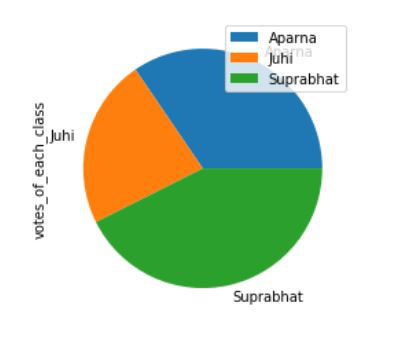## Pie Chart as Percentage

To add percentage autopct attribute is set to an appropriate value, this automatically adds percentages to each section.

Syntax:

`plot(kind='pie', autopct)`

Example: Adding percentages to pie chart

## Python3

 `import` `pandas as pd`` ` `# DataFrame of each student and the votes they get``dataframe ``=` `pd.DataFrame({``'Name'``: [``'Aparna'``, ``'Aparna'``, ``'Aparna'``, ``                                   ``'Aparna'``, ``'Aparna'``, ``'Juhi'``, ``                                   ``'Juhi'``, ``'Juhi'``, ``'Juhi'``, ``'Juhi'``,``                                   ``'Suprabhat'``, ``'Suprabhat'``, ``                                   ``'Suprabhat'``, ``'Suprabhat'``, ``                                   ``'Suprabhat'``],``                          ``'votes_of_each_class'``: [``12``, ``9``, ``17``, ``19``, ``                                                  ``20``, ``11``, ``15``, ``12``, ``                                                  ``9``, ``4``, ``22``, ``19``, ``17``, ``                                                  ``19``, ``18``]})`` ` `# Plotting the pie chart for above dataframe``dataframe.groupby([``'Name'``]).``sum``().plot(``    ``kind``=``'pie'``, y``=``'votes_of_each_class'``, autopct``=``'%1.0f%%'``)`

Output: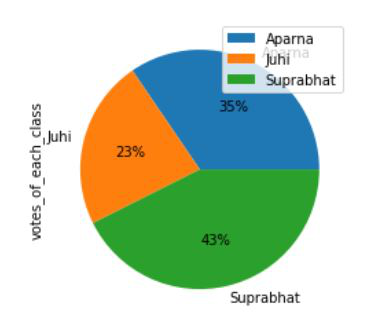## Defining Colors in a Pie Chart

To add colors to a pie chart, the colors attribute is set to an appropriate list of colors.

Syntax:

`plot(kind='pie', colors)`

Example: Adding colors to pie chart

## Python3

 `import` `pandas as pd`` ` `# DataFrame of each student and the votes they get``dataframe ``=` `pd.DataFrame({``'Name'``: [``'Aparna'``, ``'Aparna'``, ``'Aparna'``, ``                                   ``'Aparna'``, ``'Aparna'``, ``'Juhi'``,``                                   ``'Juhi'``, ``'Juhi'``, ``'Juhi'``, ``'Juhi'``,``                                   ``'Suprabhat'``, ``'Suprabhat'``, ``                                   ``'Suprabhat'``, ``'Suprabhat'``, ``                                   ``'Suprabhat'``],``                          ``'votes_of_each_class'``: [``12``, ``9``, ``17``, ``19``,``                                                  ``20``, ``11``, ``15``, ``12``, ``                                                  ``9``, ``4``, ``22``, ``19``, ``17``,``                                                  ``19``, ``18``]})`` ` `# Defining colors for the pie chart``colors ``=` `[``'pink'``, ``'silver'``, ``'steelblue'``]`` ` `# Plotting the pie chart for above dataframe``dataframe.groupby([``'Name'``]).``sum``().plot(``    ``kind``=``'pie'``, y``=``'votes_of_each_class'``, ``  ``autopct``=``'%1.0f%%'``, colors``=``colors)`

Output: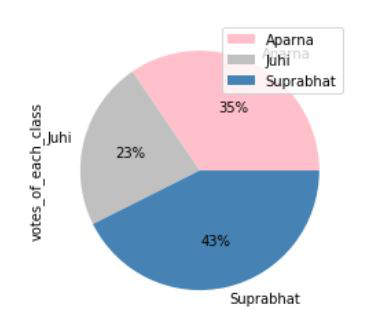## Explode effect in Pie Chart

Exploding a pie chart means breaking it into its segments. For this, we use explode attribute and set it to an appropriate value.

Syntax:

`plot(kind='pie', explode)`

Example: Exploding a pie chart

## Python3

 `import` `pandas as pd`` ` `# DataFrame of each student and the votes they get``dataframe ``=` `pd.DataFrame({``'Name'``: [``'Aparna'``, ``'Aparna'``, ``'Aparna'``, ``                                   ``'Aparna'``, ``'Aparna'``, ``'Juhi'``, ``                                   ``'Juhi'``, ``'Juhi'``, ``'Juhi'``, ``'Juhi'``,``                                   ``'Suprabhat'``, ``'Suprabhat'``, ``                                   ``'Suprabhat'``, ``'Suprabhat'``, ``                                   ``'Suprabhat'``],``                          ``'votes_of_each_class'``: [``12``, ``9``, ``17``, ``19``, ``                                                  ``20``, ``11``, ``15``, ``12``,``                                                  ``9``, ``4``, ``22``, ``19``, ``17``, ``                                                  ``19``, ``18``]})`` ` `# Defining colors for the pie chart``colors ``=` `[``'pink'``, ``'silver'``, ``'steelblue'``]`` ` `# Define the ratio of gap of each fragment in a tuple``explode ``=` `(``0.05``, ``0.05``, ``0.05``)`` ` `# Plotting the pie chart for above dataframe``dataframe.groupby([``'Name'``]).``sum``().plot(``    ``kind``=``'pie'``, y``=``'votes_of_each_class'``, autopct``=``'%1.0f%%'``,``  ``colors``=``colors, explode``=``explode)`

Output :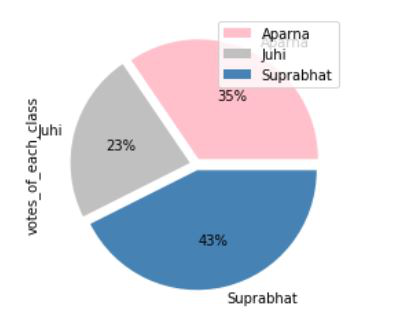## Shadow effect in Pie Chart

Shadow adds an extra dimension to our pie chart, for this just set the shadow attribute to True.

Syntax:

`plot(kind='pie', shadow=True)`

Example: Shadow effect in pie chart

## Python3

 `import` `pandas as pd`` ` `# DataFrame of each student and the votes they get``dataframe ``=` `pd.DataFrame({``'Name'``: [``'Aparna'``, ``'Aparna'``, ``'Aparna'``, ``                                   ``'Aparna'``, ``'Aparna'``, ``'Juhi'``, ``                                   ``'Juhi'``, ``'Juhi'``, ``'Juhi'``, ``'Juhi'``,``                                   ``'Suprabhat'``, ``'Suprabhat'``, ``                                   ``'Suprabhat'``, ``'Suprabhat'``, ``                                   ``'Suprabhat'``],``                          ``'votes_of_each_class'``: [``12``, ``9``, ``17``, ``19``, ``                                                  ``20``, ``11``, ``15``, ``12``, ``                                                  ``9``, ``4``, ``22``, ``19``, ``17``, ``                                                  ``19``, ``18``]})`` ` ` ` `# Plotting the pie chart for above dataframe``# and implementing shadow effect``dataframe.groupby([``'Name'``]).``sum``().plot(``    ``kind``=``'pie'``, y``=``'votes_of_each_class'``, autopct``=``'%1.0f%%'``, shadow``=``True``)`

Output: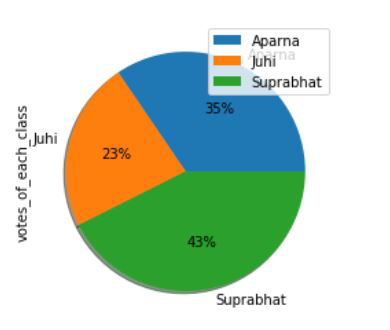## Setting Start Angle in Pie Chart

The start Angle implies that we can rotate the pie chart according to the degree angle we specified. For this startangle attribute is set to the appropriate value.

Syntax:

`plot(kind='pie', startangle)`

Example: Setting a start angle in Pie chart

## Python3

 `import` `pandas as pd`` ` `# DataFrame of each student and the votes they get``dataframe ``=` `pd.DataFrame({``'Name'``: [``'Aparna'``, ``'Aparna'``, ``'Aparna'``, ``                                   ``'Aparna'``, ``'Aparna'``, ``'Juhi'``,``                                   ``'Juhi'``, ``'Juhi'``, ``'Juhi'``, ``'Juhi'``,``                                   ``'Suprabhat'``, ``'Suprabhat'``, ``                                   ``'Suprabhat'``, ``'Suprabhat'``, ``                                   ``'Suprabhat'``],``                          ``'votes_of_each_class'``: [``12``, ``9``, ``17``, ``19``,``                                                  ``20``, ``11``, ``15``, ``12``, ``                                                  ``9``, ``4``, ``22``, ``19``, ``17``, ``                                                  ``19``, ``18``]})`` ` ` ` `# Plotting the pie chart for above dataframe``# and rotating the pie chart by 60 degrees``dataframe.groupby([``'Name'``]).``sum``().plot(``    ``kind``=``'pie'``, y``=``'votes_of_each_class'``, autopct``=``'%1.0f%%'``, startangle``=``60``)`

Output :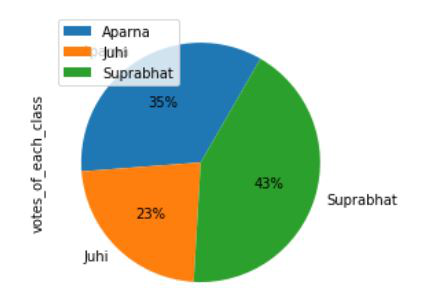My Personal Notes arrow_drop_up